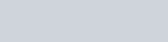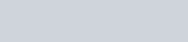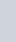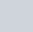The probability that a non-leap year has 53 sundays, is
Question:

The probability that a non-leap year has 53 sundays, is

(a) $\frac{2}{7}$

(b) $\frac{5}{7}$

(C) $\frac{6}{7}$

(d) $\frac{1}{7}$

Solution:

GIVEN: A non leap year

TO FIND: Probability that a non leap year has 53 Sundays.

Total number of days in non leap year is 365days

Hence number of weeks in a non leap year isIn a non leap year we have 52 complete weeks and 1 day which can be any day of the week e.g. Sunday, Monday, Tuesday, Wednesday, Thursday, Friday, and Saturday

To make 53 Sundays the additional day should be Sunday

Hence total number of days is 7

Favorable day i.e. Sunday is 1

We know that PROBABILITY =Hence probability that a non leap year has 53 Sundays isHence the correct option is